# How to Use CHOOSE to Sum or Average Cell Ranges and Replace Nested IF Statements in Excel 2007 and Excel 2010

Robbie mostly writes about Skyrim but also occasionally sheds light on the oddities of Microsoft applications such as Excel and Outlook.

In this article, I will be looking at the CHOOSE function. In its simplest form, the CHOOSE function allows you to select a value from a list. So, you could, for example, have a list of seven items (such as the Seven Dwarfs) and ask the function to return the second item in the list.

CHOOSE can be used in conjunction with other functions such as SUM or AVERAGE, so I could SUM cells between a starting cell and another cell chosen by CHOOSE from a range.

At a more advanced level, it can be used to replace nested IF statements and can be configured to choose a value based on the results of a formula or the results of a Control such as a Combo Box.

We will work through an example of each scenario to illustrate the power of this useful and versatile function. The figure below shows how CHOOSE can enable you to create much simpler and easier formulas.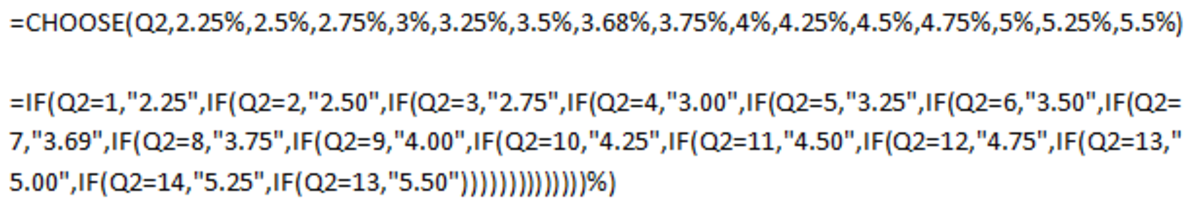An example of how using CHOOSE allows for simpler formulas (in this case compared to a formula with nested IF statements) in Excel 2007 and Excel 2010.http://robbiecwilson.hubpages.com/

## Using the CHOOSE Function in a Formula to Select an Item From a List in Excel 2007 and Excel 2010

In our first example, we will use a simple formula to use the CHOOSE function to fetch an item from a list.

• First of all, I enter my data into the cells B30 to B36.
• I then enter the following formula into C30

=CHOOSE(2,B30,B31,B32,B33,B34,B35,B36)

The formula is made up of two parts:

• Which item in the list we are selecting (in this case 2)
• The cells that contain the list of values (for this example B30,B31,B32,B33,B34,B35,B36)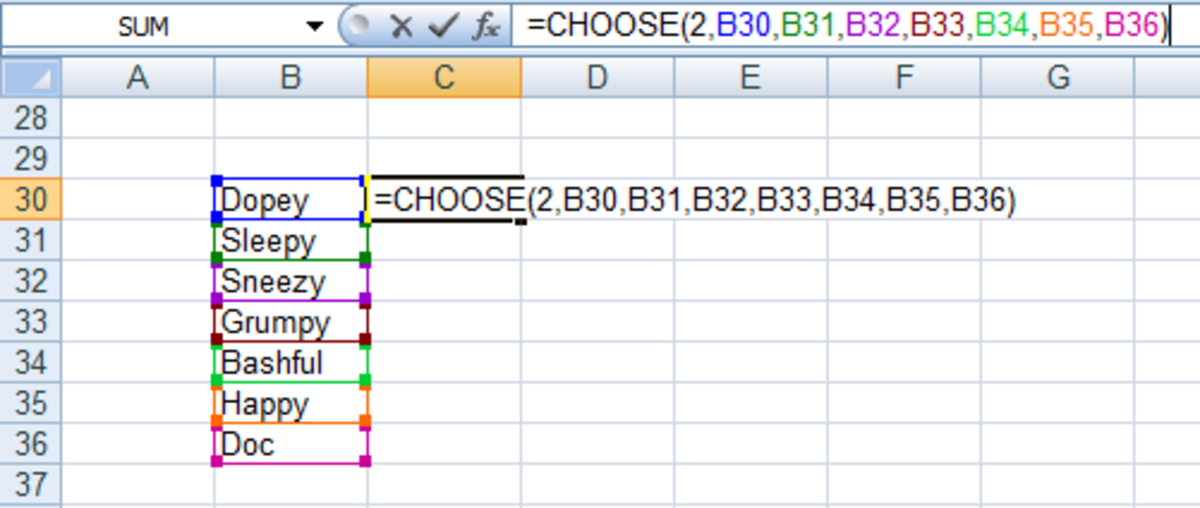Example of how to use the CHOOSE function to select an item from a list in Excel 2007 and Excel 2010.http://robbiecwilson.hubpages.com/

Note: You can have up to 254 cells containing values making up your list in your formula should you wish to.

In this instance, the formula returns the contents of the cell B31 as the answer which is Sleepy.

Note: If I change the contents of the B31, Excel will automatically change the result of our formula in C30.

## Using CHOOSE With SUM in a Formula to Sum a Range of Cells Using Excel 2007 or Excel 2010

In our next example, we will use CHOOSE and SUM together to add up a range of cells.

Scroll to Continue

For our example, we have a range of numbers in the cells B38 to B44. We use the following formula to add up a subset of the range.

=SUM(CHOOSE(2,B38:B40,B40:B42,B42:B44))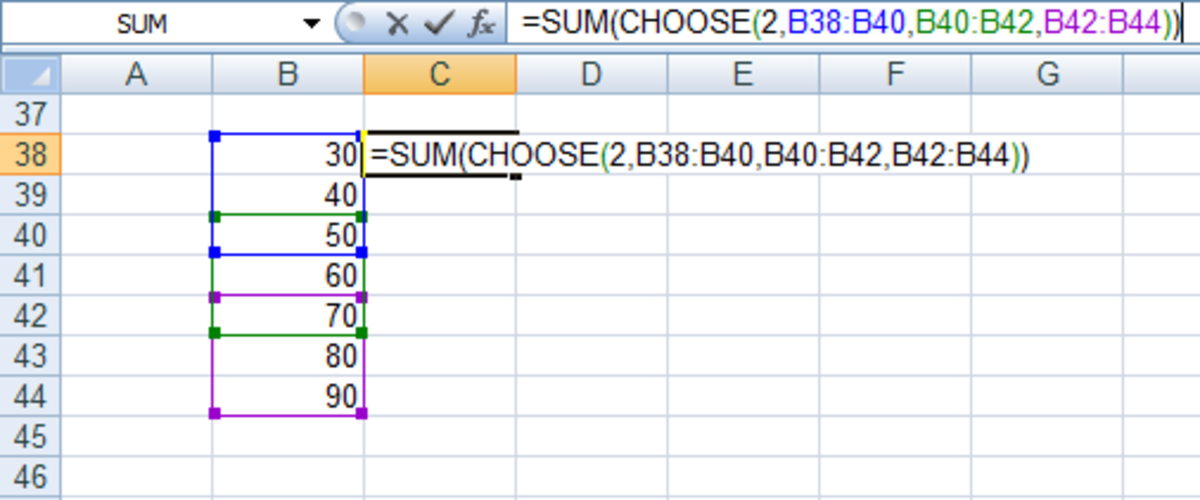An example of how to use SUM and CHOOSE together to add up a range of cells in Excel 2007 and Excel 2010.http://robbiecwilson.hubpages.com/

To understand the formula better, let’s break it down into its individual parts:

• First, as above, CHOOSE selects the 2nd item from our list which is in this case B40:B42
• Excel then uses SUM to add up the cells B40:B42

Note: The item that we are looking for does not have to be a number. It can be a reference to a cell that contains a number, or a formula that generates a number.

To illustrate this, we can use the cell reference A38 as what we are looking for and enter 1 into cell A38. This allows you to easily change what we are asking CHOOSE to fetch for us without making any changes to the formula itself.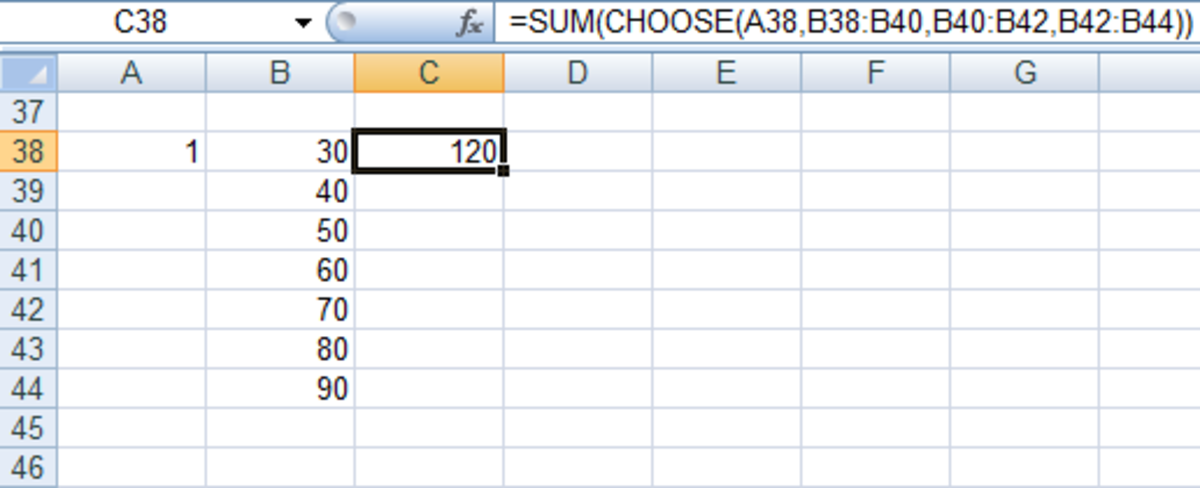An example of how to use the CHOOSE function with cell references in Excel 2007 and Excel 2010.http://robbiecwilson.hubpages.com/

The formula becomes:

=SUM(CHOOSE(A38,B38:B40,B40:B42,B42:B44))

Note: If I enter a number into A38 that is outside the range of the CHOOSE function in our formula (so in this example 4 as there is no fourth item to choose from), Excel will display a #VALUE error rather than an answer.

## Using the CHOOSE Function to Replace Nested IF Statements and Using CHOOSE With Combo Boxes in Excel 2007 and Excel 2010

Now that we have shown how to use CHOOSE to select an item from a list and also how to use it in conjunction with other functions such as SUM, we will illustrate how to use it to replace nested IF statements and also how to use it with Combo boxes.

For this example, I will use the panel I made from an article I wrote on how to create a Mortgage calculator. It used a range of functions including PMT, PPMT, IPMT and ABS as well as also using Combo boxes to allow users of my spreadsheet to select their interest rate and mortgage length from a drop-down list and have their repayments automatically calculated.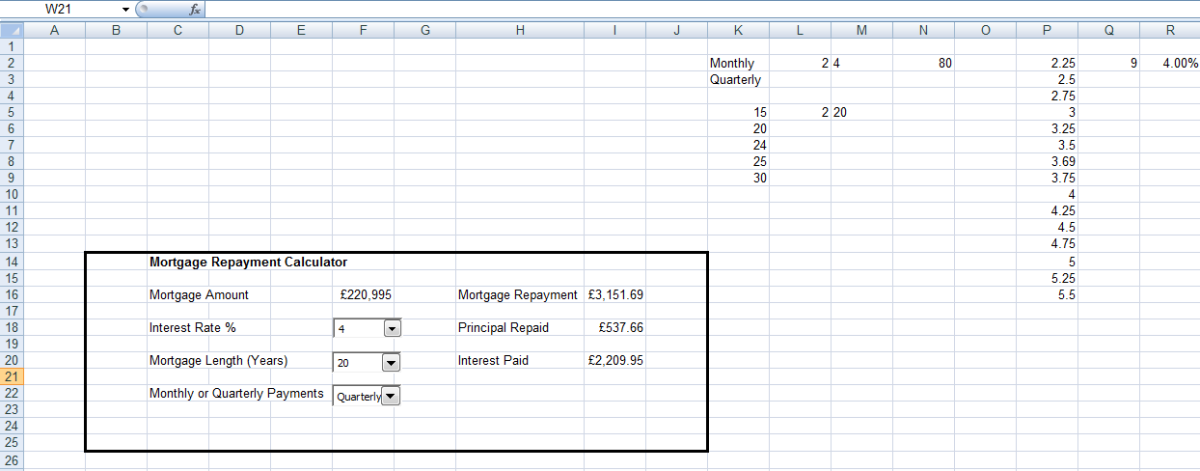User panel created for my Mortgage Repayment Calculator to be used to illustrate replacing nested IF statements with CHOOSE in Excel 2007 and Excel 2010.http://robbiecwilson.hubpages.com/
• We are interested in this case in the Interest Rate that the user selects from the Combo box in cell F18.
• This Combo box allows the user to select from a list (the Combo Box uses the data contained in cells P2 to P16 to populate the drop-down)
• When a user selects an Interest Rate, Excel puts the result into cell Q2 (the Combo box is linked to cell Q2)
• You can see from the above figure that Excel enters 9 into cell Q2 when the user selects 4 from the Combo Box (as 4 is the ninth item on the list (P2 to P16))

OK, now where we need CHOOSE to help us is to convert this result in Q2 into something that we can use in our formulas which create the actual calculator. Originally, I used nested IF functions to create the following formula to convert the result of the Combo Box into an Interest Rate percentage in R2:

=IF(Q2=1,"2.25",IF(Q2=2,"2.50",IF(Q2=3,"2.75",IF(Q2=4,"3.00",IF(Q2=5,"3.25",IF(Q2=6,"3.50",IF(Q2=7,"3.69",IF(Q2=8,"3.75",IF(Q2=9,"4.00",IF(Q2=10,"4.25",IF(Q2=11,"4.50",IF(Q2=12,"4.75",IF(Q2=13,"5.00",IF(Q2=14,"5.25",IF(Q2=13,"5.50"))))))))))))))%)

What the formula does is:

• IF Q2 equals 1, then display 2.25% in R2,
• IF Q2 equals 2 then display 2.50% and so on.

We can use CHOOSE to create a much simpler and tidier formula in this case.

As we did above, we create a CHOOSE formula with two basic elements:

• Which item from the list we want Excel to choose and
• The list we want Excel to choose from

So for this formula, we want Excel to select an item from the list based on the contents of Q2 which is linked to our Combo Box in cell F18

The list is the same as listed in the Combo Box and is stored in cells P2 to P16

The formula is:

=CHOOSE(Q2,2.25%,2.5%,2.75%,3%,3.25%,3.5%,3.68%,3.75%,4%,4.25%,4.5%,4.75%,5%,5.25%,5.5%)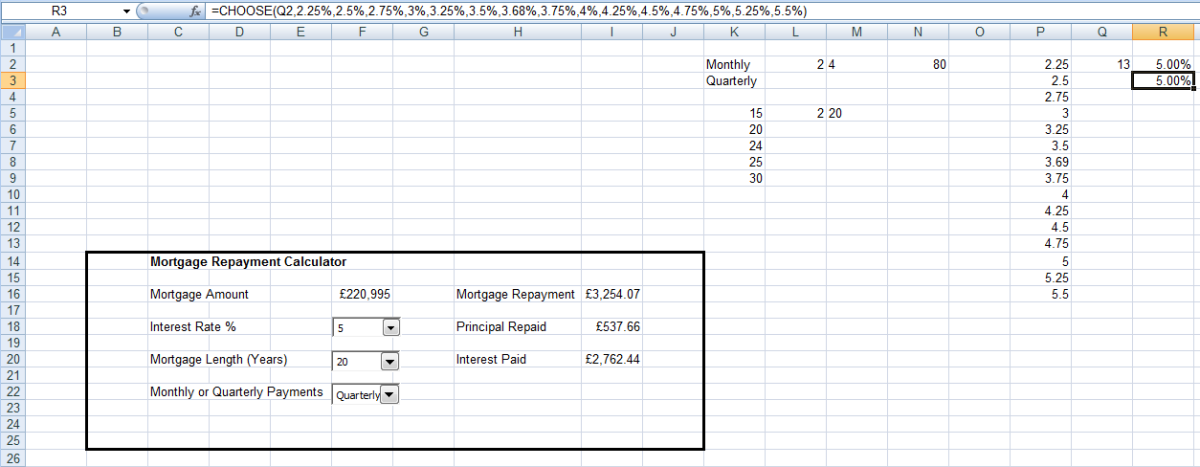Illustration of how a CHOOSE function can be used in a formula to replace multiple nested IF statements in Excel 2007 and Excel 2010.http://robbiecwilson.hubpages.com/

This is much easier to read and understand (and also to type) than the original formula which was:

=IF(Q2=1,"2.25",IF(Q2=2,"2.50",IF(Q2=3,"2.75",IF(Q2=4,"3.00",IF(Q2=5,"3.25",IF(Q2=6,"3.50",IF(Q2=7,"3.69",IF(Q2=8,"3.75",IF(Q2=9,"4.00",IF(Q2=10,"4.25",IF(Q2=11,"4.50",IF(Q2=12,"4.75",IF(Q2=13,"5.00",IF(Q2=14,"5.25",IF(Q2=13,"5.50"))))))))))))))%)

Note: Simpler formulas that achieve the same goal are always preferable as they are easier to troubleshoot if they go wrong and also easier to understand, explain and replicate.

## The CHOOSE Function Is Easy to Use

The CHOOSE function at its most simple, allows you to ask Excel to choose a specific item from a list (in my example, we chose the second dwarf from the list of the seven dwarfs). In addition, you can combine it with other functions such as SUM and AVERAGE so that Excel can sum cells from a range, for example, based on which cells you told it to add up.

Finally, we looked at more advanced uses for CHOOSE, specifically replacing nested IF statements and working with the results from Combo Boxes. This allowed us to create a shorter, more easily understood formula rather than a very long and complicated one. This helps reduce the possibility for errors and also makes your formulas easier to understand, replicate and explain.

As with all functions in Excel, the CHOOSE function has a very wide range of possible uses which is limited only by what you want it to do for you and which functions you decide to use it with. I hope that this article has been interesting and informative and you now feel comfortable using CHOOSE in formulas to assist you. Many thanks for reading, please feel free to leave any comments you may have below.

Robbie C Wilson (author) on December 12, 2013:

Hi Joel,

Thanks for your kind comment. I am so glad that you found my hub useful and that it gave you the information that you were looking for.

Joel on December 05, 2013:

Thanks Robbie! I searched the Internet for about an hour to find a solution to an Excel challenge I've been having. Your post hit the mark perfectly for me!

Regards,

Joel